# Force On Moving Charge On A Magnetic Field (Lorentz Force)

Consider a charged particle or charge =q is moving with velocity v on a magnetic field B can experience magnetic force FM. If the direction of magnetic field is represented by Y-axis, velocity of charged particle is along XY plane making angle θ with direction of magnetic field (B), then the direction of magnetic force is represented by Z-axis.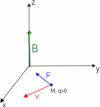Experimentally it is found that magnitude of magnetic force is
directly proportional to the magnitude of the charge i.e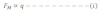directly proportional to the velocity of the charge i.e.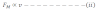directly proportional to the strength of magnetic field i.e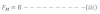directly proportional to the sine of angle between v and B i.e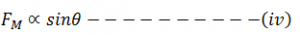Now from above equations, we get,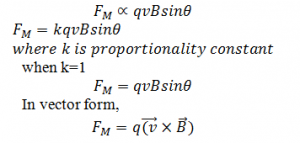This is the expression for Lorentz magnetic force where FM is perpendicular to the plane of v and B.